Courses

# Test: Two Port Network

## 15 Questions MCQ Test RRB JE for Electronics & Communication Engineering | Test: Two Port Network

Description
This mock test of Test: Two Port Network for Railways helps you for every Railways entrance exam. This contains 15 Multiple Choice Questions for Railways Test: Two Port Network (mcq) to study with solutions a complete question bank. The solved questions answers in this Test: Two Port Network quiz give you a good mix of easy questions and tough questions. Railways students definitely take this Test: Two Port Network exercise for a better result in the exam. You can find other Test: Two Port Network extra questions, long questions & short questions for Railways on EduRev as well by searching above.
QUESTION: 1

### [T] = ?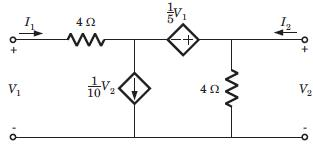​ ​ ​

Solution:

Let I3 be the clockwise loop current in center loop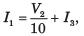V2 = 4(I2 + I3) ⇒ I3 = 0.25V2 - I2
⇒ I1 = 0.35V2 - I2  .........(i)
V1 = 4I1 - 0.2V1 + V2
1.2V1 = 4(0.35V2 - I2) + V2 = 2.4V2 - 4I2
⇒ V1 = 2V2 - 3.33I2 .........(ii)

QUESTION: 2

### [H] = ?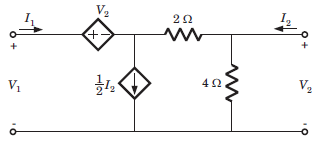​ ​ ​

Solution: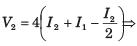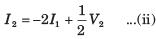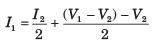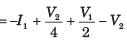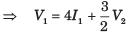..........(i)

QUESTION: 3

### [Y] = ?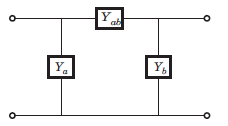​ ​ ​

Solution:

I1 = (V1 - V2) Yab + V1Yab

⇒ I1 = V1(Ya + Yab) - V2Yab .....(i)

I2 = (V2 - V1)Yab + V2Yb = -V1Yab + V2(Yb + Yab) .....(ii)

QUESTION: 4

The y-parameters of a 2-port network are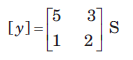A resistor of 1 ohm is connected across as shown in fig. The new y –parameter would be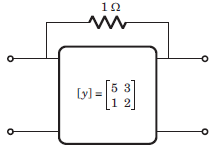​ ​ ​

Solution:

y-parameter of 1Ω resistor network are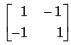New y-parameter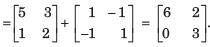QUESTION: 5

For the 2-port of fig.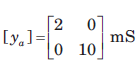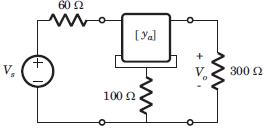The value of vo/vs is

Solution: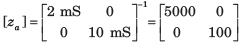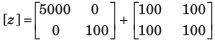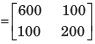V1 = 600I1 + 100I2 , V2 = 100I1 + 200I2
Vs = 60I1 + V1 = 660I1 + 100I2 , V2 = Vo = -300I2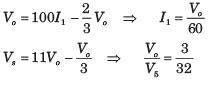QUESTION: 6

The T-parameters of a 2-port network are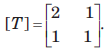If such two 2-port network are cascaded, the z –parameter for the cascaded network is

Solution: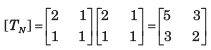​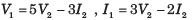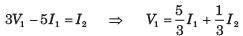.......(i)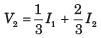........(ii)

QUESTION: 7

[Y] = ?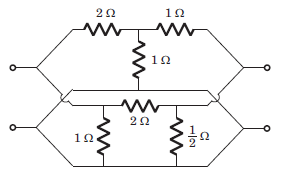​ ​ ​

Solution: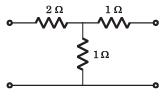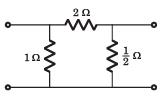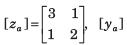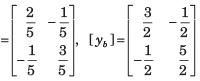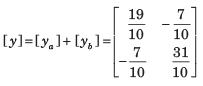QUESTION: 8

Which among the following is regarded as short circuit forward transfer admittance?

Solution:
QUESTION: 9

h21 = ?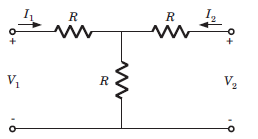Solution: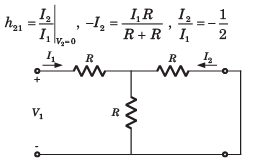QUESTION: 10

In the circuit shown in fig. P.1.10.34, when the voltage V1 is 10 V, the current I is 1 A. If the applied voltage at port-2 is 100 V, the short circuit current flowing through at port 1 will be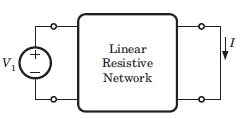Solution: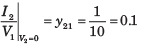Interchaning the port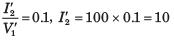QUESTION: 11

For a 2-port symmetrical bilateral network, iftransmission parameters A = 3 and B = 1Ω , the value ofparameter C is

Solution:

For symmetrical network A = D = 3

For bilateral AD - BC = 1, 9 - C = 1,C = 8 S

QUESTION: 12

A network has 7 nodes and 5 independent loops. The number of branches in the network is

Solution:
QUESTION: 13

The circuit shown in fig. is reciprocal if a is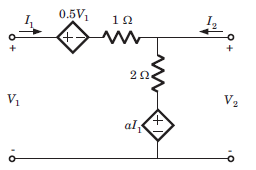Solution:

V1 = 0.5V1 + I1 + 2(I1 + I2) + aI1
⇒ V1 = (6 + 2a)I1 + 4I2 ......(i)
V2 = 2(I1 + I2) + aI1 ⇒ V2 = (2 + a)I1 + 2I2 ......(ii)
For reciprocal network
z12 = z21, 4 = 2 + a ⇒ a = 2

QUESTION: 14

Zin = ?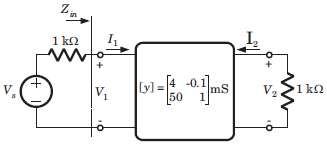Solution:

I1 = 4 x 103V1 -0.1 x 10-3V2
I2 = 50 X 10-3V1 + 10-3V2 ,V2 = -103I2

- 10-3V2 = 50 x 10-3V1 + 10-3V2, V2 = -25V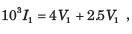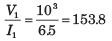QUESTION: 15

v1 ,v2 = ?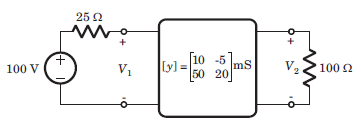Solution:

I1 = 10 x 10-3V1 -5 x 10-3V2

100 = 25I1 + V1
100 - V1 = 0.25V1 - 0.25V2 ⇒ 800 = 10V1 - V2   ....(i)
I2 = 50 x 10-3V1 + 20 x 10-3V2, V2 = -100I2
V2 = -5V1 -V2 ⇒ 3V2 + 5V1 = 0  ....(ii)
From (i) and (ii) V1 = 68.6 V, V2 = -114.3 V.